# ISEE Lower Level Math : Geometry

## Example Questions

### Example Question #51 : How To Find The Perimeter Of A Triangle

Find the length of one side of an equilateral triangle that has a perimeter of 27cm.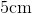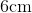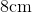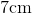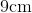Explanation:

The formula to find perimeter of an equilateral triangle is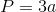where a is the length of one side.  We can multiply that by 3, because an equilateral triangle has 3 equal sides.  Now, to find the length of one side, we will solve for a.

So, we know the perimeter of the equilateral triangle is 27cm.  Knowing this, we can substitute.  We get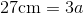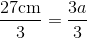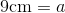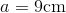Therefore, the length of one side of the equilateral triangle is 9cm.

### Example Question #1321 : Isee Lower Level (Grades 5 6) Mathematics Achievement

Use the following triangle to answer the question: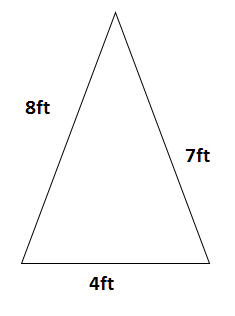Find the perimeter.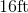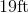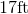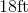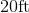Explanation:

To find the perimeter of a triangle, we will use the following formula: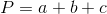where a, b, and c are the lengths of the sides of the triangle.

Now, given the trianglewe can see that it has sides of length 8ft, 4ft, and 7ft.  So, we get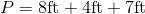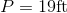### Example Question #1 : How To Find Symmetry

There is a four sided figure in which none of the lines run parallel to each other. Which of the following could be the appropriate term to describe the figure?

Trapezoid

None of these

Rectangle

Parallelogram

Explanation:

A key characteristic of a rectangle, parallelogram, and trapezoid is the fact that they each have at least one pair of lines that run parallel to each other. The only option that has lines that may not run parallel to each other is the quadrilateral, which must simply have four sides but has no specifications about parallelism.

### Example Question #1 : Lines

If a diagonal is drawn from one corner of a rectangle to the opposite corner, what 2 shapes result?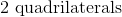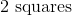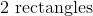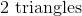Explanation:

While drawing a line across a rectangle (so that it bisects 2 sides) can result in 2 quadrilaterals, squares, or rectangles, a line drawn from one corner to the furthest corner results in two triangles. Therefore, the correct answer choice is 2 triangles.

### Example Question #2 : How To Find Symmetry

How many lines of symmetry are there in a square?Explanation:

A line of symmetry is a line that divides a polygon in half and each half is a mirror image of the other. In other words, you can fold the polygon over the symmetry line and each half matches up perfectly.

For a square there are four lines of symmetry. Two are from the diagonals of the square and two are from connecting the midpoints of the opposite sides.

### Example Question #1 : Lines

What is the distance between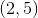and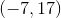?Explanation:

The distance formula is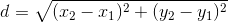.

Let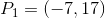and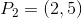: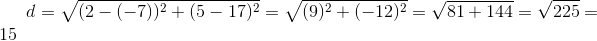### Example Question #372 : Plane Geometry

What is the distance between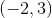and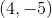?Explanation:

The distance formula is given by.

Let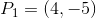and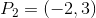: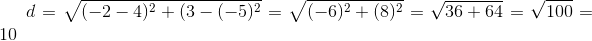### Example Question #1 : How To Find Length Of A Line

What is the distance between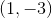and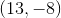?Explanation:

The distance formula is given by.

Let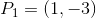and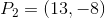: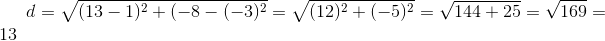### Example Question #1 : How To Find Length Of A Line

What is the distance between the points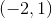and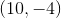?Explanation:

The distance formula is.

Let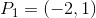and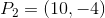.

Plug these two points into the distance formula: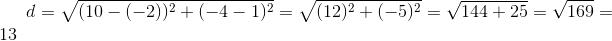### Example Question #1 : Lines

If a line with a length of 5 starts at the point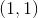, which of the following is NOT a possible end point?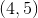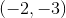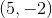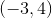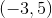Explanation:

Let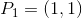, and let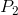be the end point we are looking for.

Recall the distance formula:Squaring the distance formula gives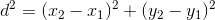.

Plugthis squared distance formula and solve for: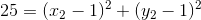Substitute each response into this equation to see which one is false.  The only response that doesn't work is.

### All ISEE Lower Level Math Resources